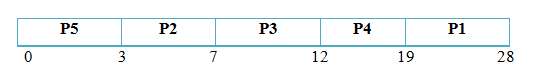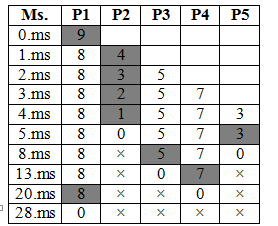• In this algorithm, the process that has the shortest CPU burst time is selected first for the execution.
• When the CPU is available it is assign to the process that has the smallest next CPU burst.
• If two processes have the same length of CPU burst then FCFS policy is used to solve the tie.
• In fact more appropriate term for this algorithm would be the “Next Shortest CPU Burst Time First” because it allocates the CPU by examining the length of the next CPU burst of a process rather than its total length of a burst time.
• SJF algorithm may be either preemptive or non-preemptive.
• When a new process arrives having a short CPU burst time than the currently executing process; preemptive SJF algorithm will preempt the currently executing process, and allocates the CPU to the newly arrived process where as a non-preemptive SJF algorithm will allow the current running process to finish its CPU burst.
• Preemptive SJF scheduling is also referred to as Shortest Remaining Time Next (SRTN) scheduling.
• Les us see how this policy works for the scheduling of processes with the help of an example.

#### Non-Preemptive SJF

Consider the following processes and their CPU burst time (in millis.) and find out average waiting time and average turnaround time using non-preemptive SJF technique.

 Process Burst Time (mills.) P1 9 P2 4 P3 5 P4 7 P5 3 Total 28
• As per the rule of Shortest Job First CPU will be allocated to the process with the shortest CPU burst time so in our example, process P5 has the shortest CPU burst time so obviously process P5 will get the CPU first for execution thus waiting time for P1 will be zero.
• After that process P2 has the smallest CPU burst so P2 will get next tern after completion of P1 so process P2 has to wait for 3 milliseconds which is the completion time for process P5.
• Next turn is for process P3 with shortest burst time 5 milliseconds. waiting time for process P3 will be calculated as 3 + 4 = 7 (burst time of P5 and P2). It can be represented in the gantt chart as follow:Total Waiting Time:

``````P1 = 19 + P2 = 3 + P3 = 7 (3+4) + P4 = 12 (3+4+5) + P5 = 0 (ms)
= 41 mills.``````

Avg. Waiting Time :

``````Avg. Waiting Time =  Total Waiting Time / No.of Process

= 41 / 5

= 8.2 mills.``````

Total Turnaround Time :

``````P1 = 28 + P2 = 7 + P3 = 12 + P4 = 19 + P5 = 3

= 69 mills.``````

Avg. Turnaround Time :

``````Avg. Turnaround Time  = Total TurnaroundTime / No.of Process

= 69/5

= 13.8 mills.``````

#### Preemptive SJF

Consider the following processes and their CPU burst time (in millis.) and find out average waiting time and average turnaround time using preemptive SJF technique.

 Process Burst Time (ms.) Arrival Time P1 9 0 P2 4 1 P3 5 2 P4 7 3 P5 3 4 Total 28
• Notice that all processes arrives at different time period so we also need to consider their arrival time while finding shortest CPU burst from the available processes.
• So if we look to the arrival order of processes, P1 is the first process as its arrival time is zero. At zero millisecond we have only one process available in ready queue i.e. P1 so obviously process P1 will get the CPU first as we have no choice for comparison.
• After one millisecond a new process enter into the ready queue i.e process P2 with burst time of 4 milliseconds. The execution of currently running process P1 is completed of about one milliseconds when new process P2 arrives. so now we have total two processes into the ready queue for selection based on shortest CPU burst time.
• After one millisecond the burst time of process P1 is 8  (9-1 millis.) and process P2 with burst time 4. Since P2 has shortest CPU burst time, execution of currently running process P1 will be stopped by deallocating the CPU from P1 and P2 will be selected for execution by allocating the CPU to it.
• Similarly, at the time of two milliseconds, another new process P3 with burst time of 5 milliseconds arrives into the ready queue. Current execution of P2 is one milliseconds and remaining burst time is 3 milliseconds (4-1).
• Now we have total three processes into the ready queue so again their burst time will be compared and process having shortest burst time will be selected. In our case remaining burst time of process P1 is 8, P2 has 3 and P3 with 5 milliseconds respectively. Process P2 will continue to execute as it has the shortest burst time.
• In similar fashion, whenever a new process arrives, their burst time will be compared to find out process with the minimum burst time for selection.
• The complete sequence can be represented as below:#### Waiting Time

``````Waiting Time =Total waiting Time – No.of Milisec. Process executed – Arrival Time

P1 = 20 – 1 – 0 = 19 ms,

P2 = 4 – 3 – 1 = 0 ms,

P3 = 8 - 0 – 2 = 6ms

P4 = 13 – 0 – 3 = 10 ms,

P5 = 5 - 0 -  4 =  1 ms.

Total Waiting Time = 36 mills.``````

### Avg.Waiting Time:

``36 / 5  =  7.2 mills``

### Turnaround Time

``````Turnaround Time = Total Turnaround Time- Arrival Time

P1 = 28 – 0 =28 ms,

P2 = 5 – 1 = 4,

P3 = 13 – 2 = 11,

P4 = 20 – 3 = 17,

P5 = 8 – 4 = 4

Total Turnaround Time= 64 mills.
``````

### Average Turnaround Time

``````Avg. Turnaround Time  = Total Turnaround Time / No.of Process
= 64 / 5

= 12.8 mills.    ``````